# ISEE Upper Level Quantitative : How to find the volume of a sphere

## Example Questions

### Example Question #333 : Geometry

In terms of, give the volume, in cubic feet, of a spherical tank with diameter 36 inches.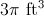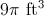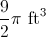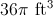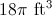Explanation:

36 inches =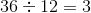feet, the diameter of the tank. Half of this, orfeet, is the radius. Set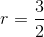, substitute in the volume formula, and solve for: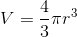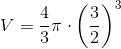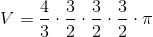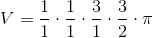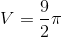### Example Question #1 : How To Find The Volume Of A Sphere

Which is the greater quantity?

(a) The volume of a sphere with radius(b) The volume of a cube with sidelength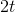It is impossible to tell from the information given

(a) and (b) are equal

(a) is greater

(b) is greater

(b) is greater

Explanation:

A sphere with radiushas diameterand can be inscribed inside a cube of sidelength. Therefore, the cube in (b) has the greater volume.

### Example Question #2 : How To Find The Volume Of A Sphere

Which is the greater quantity?

(a) The volume of a cube with sidelengthinches.

(b) The volume of a sphere with radiusinches.

(a) and (b) are equal.

(b) is greater.

It is impossible to tell from the information given.

(a) is greater.

(a) is greater.

Explanation:

You do not need to calculate the volumes of the figures. All you need to do is observe that a sphere with radiusinches has diameterinches, and can therefore be inscribed inside the cube with sidelengthinches. This give the cube larger volume, making (a) the greater quantity.

### Example Question #3 : How To Find The Volume Of A Sphere

Which is the greater quantity?

(a) The volume of a sphere with diameter one foot

(b)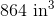(a) and (b) are equal.

(b) is greater.

It is impossible to tell from the information given.

(a) is greater.

(a) is greater.

Explanation:

The radius of the sphere is one half of its diameter of one foot, which is six inches, so substitute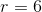: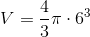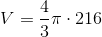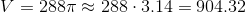cubic inches,

which is greater than.

### Example Question #4 : How To Find The Volume Of A Sphereis a positive number. Which is the greater quantity?

(A) The volume of a cube with edges of length(B) The volume of a sphere with radiusIt is impossible to determine which is greater from the information given

(A) and (B) are equal

(B) is greater

(A) is greater

(A) is greater

Explanation:

No calculation is really needed here, as a sphere with radius- and, subsequently, diameter- can be inscribed inside a cube of sidelength. This makes (A), the volume of the cube, the greater.

### Example Question #2 : Spheres

Which is the greater quantity?

(a) The radius of a sphere with surface area(b) The radius of a sphere with volume(a) and (b) are equal

(b) is the greater quantity

It cannot be determined which of (a) and (b) is greater

(a) is the greater quantity

(a) and (b) are equal

Explanation:

The formula for the surface area of a sphere, given its radius, is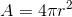The sphere in (a) has surface area, so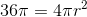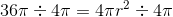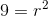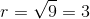The formula for the volume of a sphere, given its radius, isThe sphere in (b) has volume, so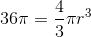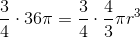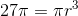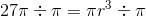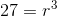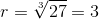The radius of both spheres is 3.